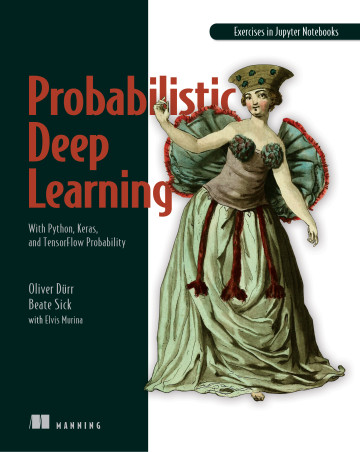</a>

# Notebooks overview

You can use the notebooks below by clicking on the Colab Notebooks link or running them locally on your machine.

To run them locally, you can either

## Chapter 2: Neural network architectures

Number Topic Github Colab
1 Banknote classification with fcNN nb_ch02_01 nb_ch02_01
2 MNIST digit classification with shuffling nb_ch02_02 nb_ch02_02
2a MNIST digit classification with fcNN nb_ch02_02a nb_ch02_02a
3 CNN edge lover nb_ch02_03 nb_ch02_03
4 Causal and time dilated convolutions nb_ch02_04 nb_ch02_04

## Chapter 3: Principles of curve fitting

Number Topic Github Colab
1 Gradient descent method for linear regression with one tunable parameter nb_ch03_01 nb_ch03_01
2 Gradient descent method for linear regression nb_ch03_02 nb_ch03_02
3 Linear regression with TensorFlow nb_ch03_03 nb_ch03_03_tf2 nb_ch03_03 nb_ch03_03_tf2
4 Backpropagation by hand nb_ch03_04 nb_ch03_04_tf2 nb_ch03_04 nb_ch03_04_tf2
5 Linear regression with Keras nb_ch03_05 nb_ch03_05
6 Linear regression with TF Eager nb_ch03_06 nb_ch03_06
7 Linear regression with Autograd nb_ch03_07 nb_ch03_07

## Chapter 4: Building loss functions with the likelihood approach

Number Topic Github Colab
1 First example of the maximum likelihood principle: throwing a die nb_ch04_01 nb_ch04_01
2 Calculation of the loss function for classification nb_ch04_02 nb_ch04_02
3 Calculation of the loss function for regression nb_ch04_03 nb_ch04_03
4 Regression fit for non-linear relationships with non-constant variance nb_ch04_04 nb_ch04_04

## Chapter 5: Probabilistic deep learning models with TensorFlow Probability

Number Topic Github Colab
1 Modelling continuous data with Tensoflow Probability nb_ch05_01 nb_ch05_01
2 Modelling count data with Tensoflow Probability nb_ch05_02 nb_ch05_02

## Chapter 6: Probabilistic deep learning models in the wild

Number Topic Github Colab
1 Discretized Logistic Mixture distribution nb_ch06_01 nb_ch06_01
2 Regressions on the deer data nb_ch06_02 nb_ch06_02
3 Getting started with flows nb_ch06_03 nb_ch06_03
4 Using RealNVP nb_ch06_04 nb_ch06_04
5 Fun with glow nb_ch06_05 nb_ch06_05

## Chapter 7: Bayesian learning

Number Topic Github Colab
1 Predict images with a pretrained Imagenet network nb_ch07_01 nb_ch07_01
2 Bayes Linear Regression Brute Force vs Analytical nb_ch07_02 nb_ch07_02
3 Bayesian model for a coin toss nb_ch07_03 nb_ch07_03
4 Play with the analytical Bayes solution for linear regression nb_ch07_04 nb_ch07_04

## Chapter 8: Bayesian neural networks

Number Topic Github Colab
1 Linear Regression the Bayesian way nb_ch08_01 nb_ch08_01
2 Dropout to fight overfitting nb_ch08_02 nb_ch08_02
3 Regression case study with Bayesian Neural Networks nb_ch08_03 nb_ch08_03
4 Classification case study with novel class nb_ch08_04 nb_ch08_04